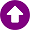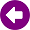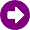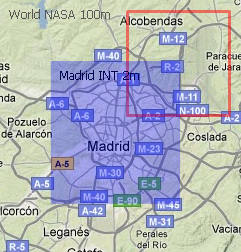﻿ Payment Calculations > Cartography Price

# CARTOGRAPHY PRICE

Navegación:  Payment Calculations > Cartography Price

# CARTOGRAPHY PRICEBesides the cost of calculating a study, there is an additional cost associated with the cartography used. The cartography price is calculated by adding the individual costs of each cartography layer. This cost is calculated based on: •Calculation cost. This cost depends on the study type and the calculation resolution•Layer use. Percentage on the profile/area of calculation which is within the layer. For example, a profile that runs half of it on a cartography layer, and half out of it, represents a 50% of use percentage. That is, the final cost of cartography layer will be half that if the profile is completely within the layer.•Layer cost. Percentage on the study cost. For example, if the layer cost is 20%, in a study of 20 euros the final layer cost is 4 euros•Individual costs of cartography = Calculation cost · Use percentage · Increase on the costThe following image is an example of getting the cartography cost. The calculation is made with two cartography layers: 100m resolution worldwide, and 2m resolution of Madrid city. The latter is shaded blue to be differentiatedThe coverage study makes calculations using 100% of worldwide cartography and 25% of the Madrid cartography within the calculation area defined by the user. The cost increment of worldwide layer is 0% (free) while the layer of Madrid city is 20%. Assuming that the calculation cost is 200 euros, cartography cost would be: •Cartography Price = 200 x 100% x 0% + 200 x 25% x 20% = 10 eurosIf instead of having used cartography of the world (for free), a rural cartography with higher resolution was used, with a cost increase of 10%, the cost would have been: •Cartography Price = 200 x 100% x 10% + 200 x 25% x 20% = 30 euros